Chrome for Android fix.

# How To Avoid Reinventing The WheelAlain Schlesser
Software Engineer & WordPress Consultant

People think that computer science is the art of geniuses.

But the actual reality is the opposite, just many people doing things that build on each other, like a wall of mini stones.

# A History Lesson

### "The Mother Of All Demos"

• Hardware + software system called "oN-Line System" (NLS)

• Mouse-driven windows-based GUI

• Video conferencing

• Revision control

• Collaborative real-time text editor

# 1968

Presented on December 9th, 1968 by Douglas Engelbert
at Computer Society's Fall Joint Computer Conference in San Francisco

### Simula

• Classes & Objects

• Subclasses and Inheritance

• Virtual Procedures (Abstract Methods)

• Coroutines (Generators)

• Garbage Collection (Automatic Memory Management)

# 1967

Presented in May 1967 by Ole-Johan Dahl and Kristen Nygaard
at the IFIP Working Conference on simulation languages in Oslo

# Wow, that's great!

## So, how are we progressing so far?

Computer Science is embarrassed by the computer.

# Basic Notions

### Building Blocks

• Algorithms

Unambiguous specifications of how to solve a class of problems.

• Data Structures

Particular way of organizing and storing data in a computer so that it can be accessed and modified efficiently.

• Multiple algorithms can solve a same problem

• Multiple data structures can be used to base these algorithms on

• Processing Speed <=> Memory Consumption

The most comon trade-off that most algorithms and data structures are measured against

### Asymptotic Notation

• Also known as Bachmann-Landau Notation, or "Big O Notation"

• f(n) = O(g(n))

"Big O(micron)" — upper bound => worst case

• f(n) = Ω(g(n))

"Big Omega" — lower bound => best case

• f(n) = Θ(g(n))

"Big Theta" — upper & lower bound => "average" case

• Usually abbreviated in Computer Science

i.e. using "O(n)" to state that an algorithm scales linearlySource: http://bigocheatsheet.com/

### Sorting Algorithms

Quicksort Mergesort Timsort Heapsort Bubble Sort Algorithm Time Complexity Space Complexity Best Average Worst Worst Ω(n log(n)) Ω(n log(n)) O(n^2) O(log(n)) Ω(n log(n)) Ω(n log(n)) O(n log(n)) O(n) Ω(n) Ω(n log(n)) O(n log(n)) O(n) Ω(n log(n)) Ω(n log(n)) O(n log(n)) O(1) Ω(n) Θ(n^2) O(n^2) O(1) Ω(n) Θ(n^2) O(n^2) O(1) Ω(n^2) Θ(n^2) O(n^2) O(1) Ω(n log(n)) Ω(n log(n)) O(n^2) O(n) Ω(n log(n)) Θ(n(log(n))^2) O(n(log(n))^2) O(1) Ω(n+k) Θ(n+k) O(n^2) O(n) Ω(nk) Θ(nk) O(nk) O(n+k) Ω(n+k) Θ(n+k) O(n+k) O(k) Ω(n) Ω(n log(n)) O(n log(n)) O(n)

Source: http://bigocheatsheet.com/

### Data Structures

Array Stack Queue Single-Linked List Double-Linked List Skip List Hash Table Binary Search Tree Cartesian Tree B-Tree Data Structure Time Complexity Space Complexity Average Worst Worst Access Search Insertion Deletion Access Search Insertion Deletion Θ(1) Θ(n) Θ(n) Θ(n) O(1) O(n) O(n) O(n) O(n) Θ(n) Θ(n) Θ(1) Θ(1) O(n) O(n) O(1) O(1) O(n) Θ(n) Θ(n) Θ(1) Θ(1) O(n) O(n) O(1) O(1) O(n) Θ(n) Θ(n) Θ(1) Θ(1) O(n) O(n) O(1) O(1) O(n) Θ(n) Θ(n) Θ(1) Θ(1) O(n) O(n) O(1) O(1) O(n) Θ(log(n)) Θ(log(n)) Θ(log(n)) Θ(log(n)) O(n) O(n) O(n) O(n) O(n log(n)) N/A Θ(1) Θ(1) Θ(1) N/A O(n) O(n) O(n) O(n) Θ(log(n)) Θ(log(n)) Θ(log(n)) Θ(log(n)) O(n) O(n) O(n) O(n) O(n) N/A Θ(log(n)) Θ(log(n)) Θ(log(n)) N/A O(n) O(n) O(n) O(n) Θ(log(n)) Θ(log(n)) Θ(log(n)) Θ(log(n)) O(log(n)) O(log(n)) O(log(n)) O(log(n)) O(n) Θ(log(n)) Θ(log(n)) Θ(log(n)) Θ(log(n)) O(log(n)) O(log(n)) O(log(n)) O(log(n)) O(n) N/A Θ(log(n)) Θ(log(n)) Θ(log(n)) N/A O(log(n)) O(log(n)) O(log(n)) O(n) Θ(log(n)) Θ(log(n)) Θ(log(n)) Θ(log(n)) O(log(n)) O(log(n)) O(log(n)) O(log(n)) O(n) Θ(log(n)) Θ(log(n)) Θ(log(n)) Θ(log(n)) O(n) O(n) O(n) O(n) O(n)

Source: http://bigocheatsheet.com/

# Most Problems Have Been Solved Decades Ago

### How To Solve Problems

1. Assume: Someone had that same problem before you

3. Find the algorithm that solves your problem

4. Find an implementation of the algorithm that fits your environment

### Algorithm Naming

• Mostly harmless

• Mostly harmless

• Mostly useless!

• Non-memorable Names of Author(s)

"Steinhaus–Johnson–Trotter Algorithm"

• Ridiculous Acronym

"RIPEMD-160"

• Ludicrous Combination of Both

"Cooley–Tukey FFT Algorithm"

• Ok, there are exceptions to these as well.

### Finding Algorithms

• Search for what you think the solution might be called

Example: "Text Pattern Recognition"

• Search for how you would describe the problem to solve

Example: "Discover recurring patterns in clusters of text"

• Browse categorized overviews

Example: List of algorithms on Wikipedia

• Use visual maps and flow charts

Example: Map of Data Mining Algorithms

# Practical Example

## WP-CLI suggestions after typo

### The Problem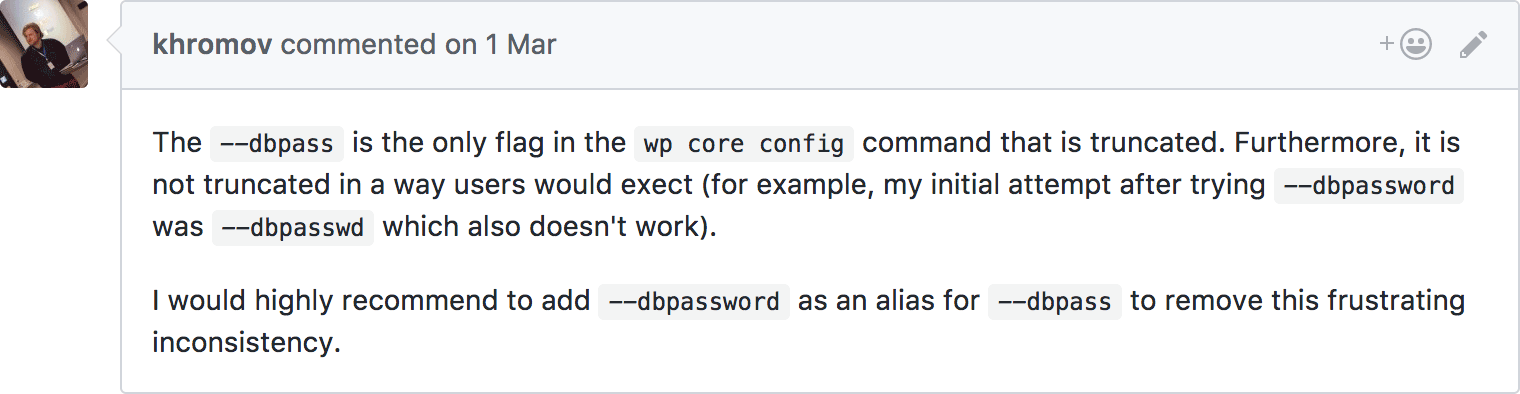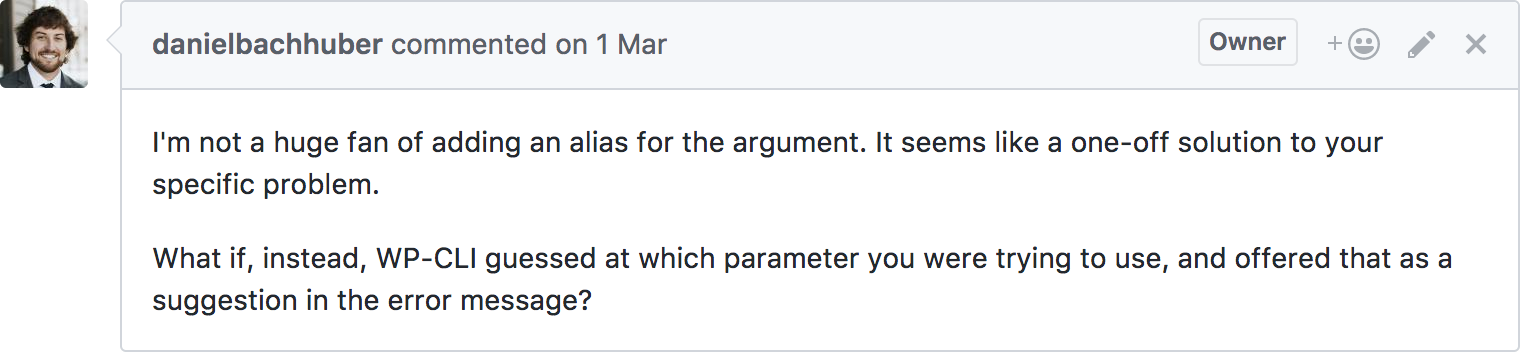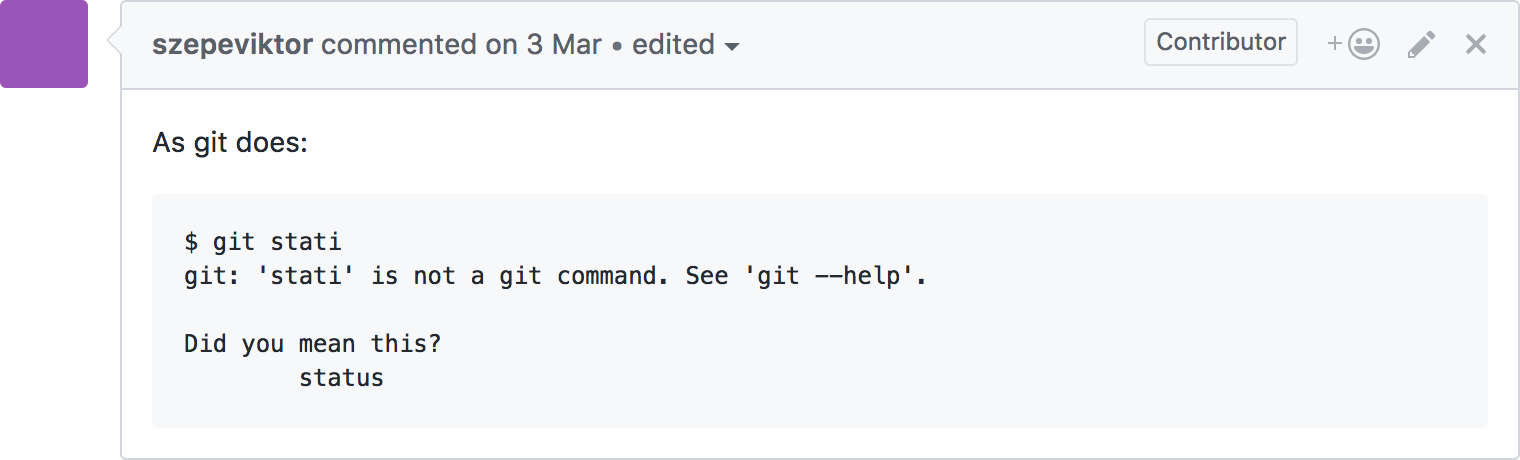### Search for an Algorithm (1/4)

• Think of a combination of nouns that describes the approach

• For the problem at hand: In a list of known arguments, find the one most similar to the user entry

• => "String Similarity"

### Search for an Algorithm (2/4)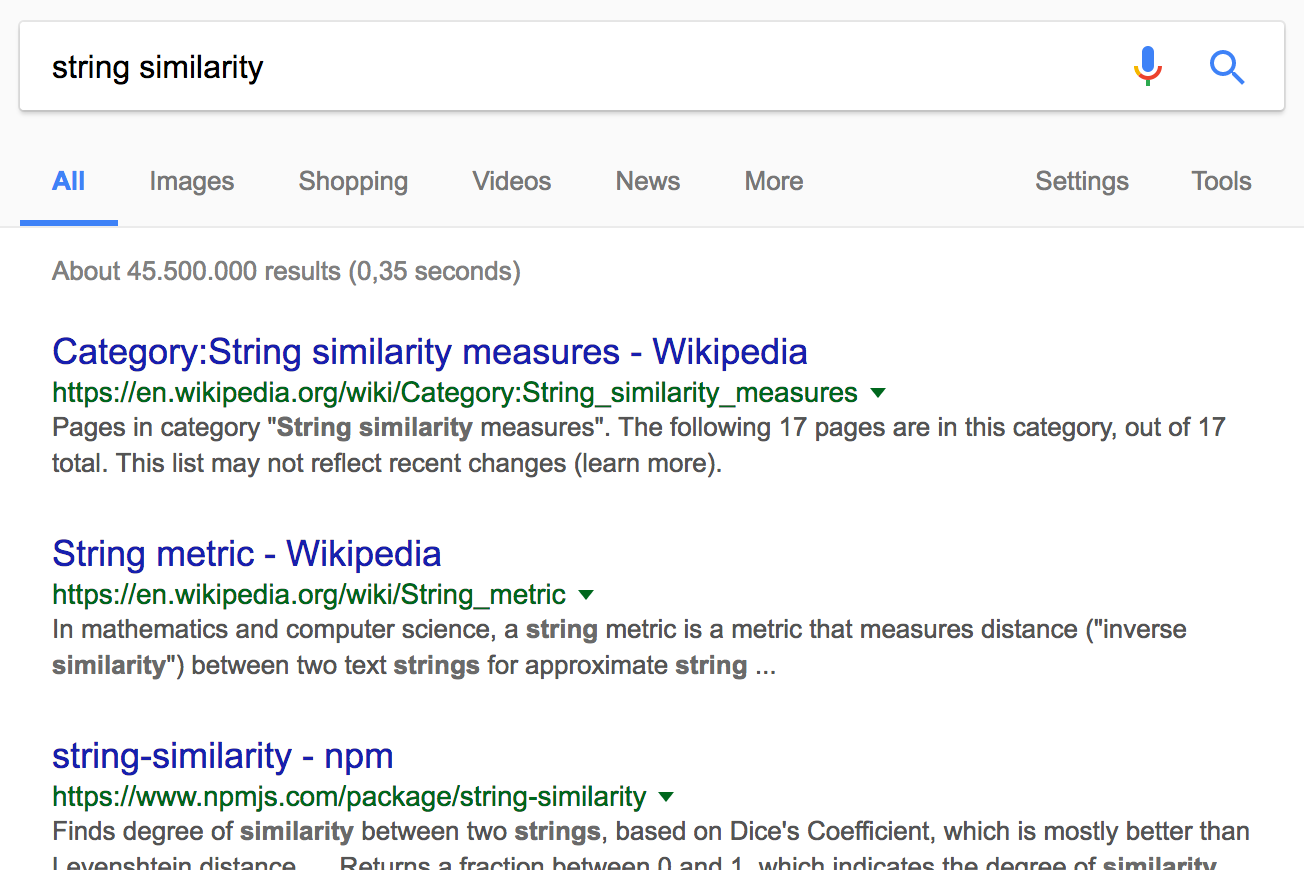### Search for an Algorithm (3/4)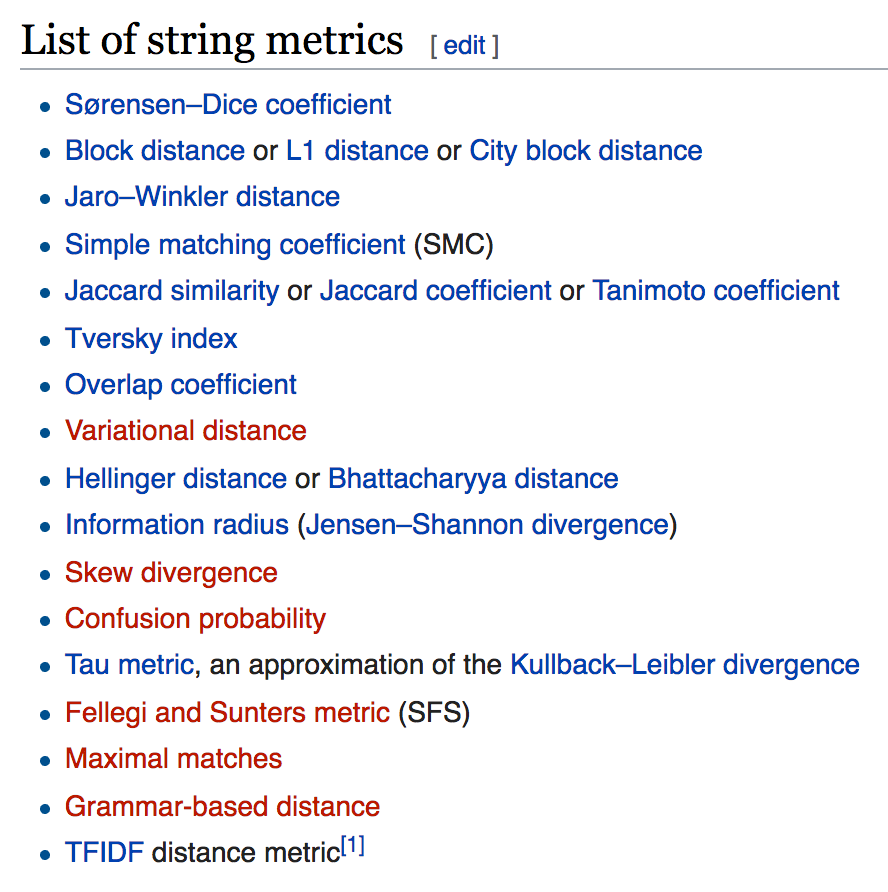### Search for an Algorithm (4/4)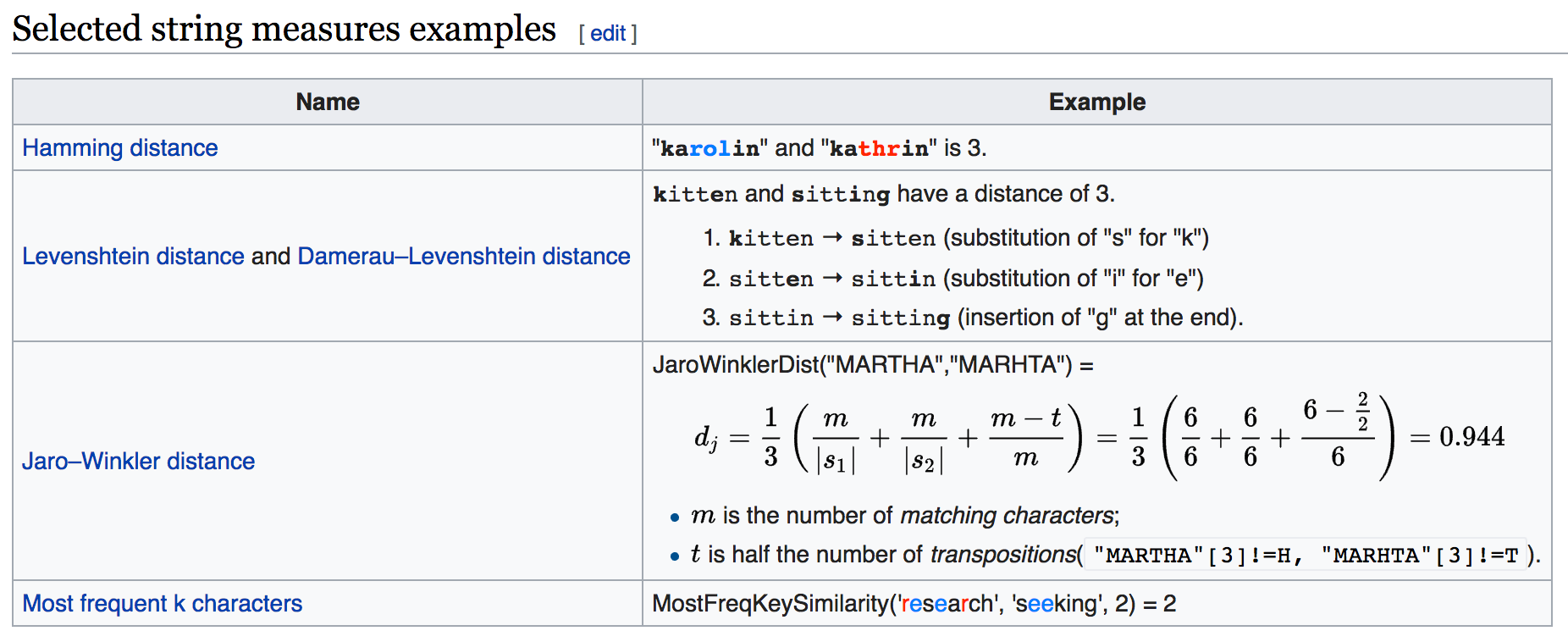### Levenshtein Distance

In information theory, Linguistics and computer science, the Levenshtein distance is a string metric for measuring the difference between two sequences.

Informally, the Levenshtein distance between two words is the minimum number of single-character edits (insertions, deletions or substitutions) required to change one word into the other.

It is named after Vladimir Levenshtein, who considered this distance in 1965.

### Levenshtein Distance

#### Definition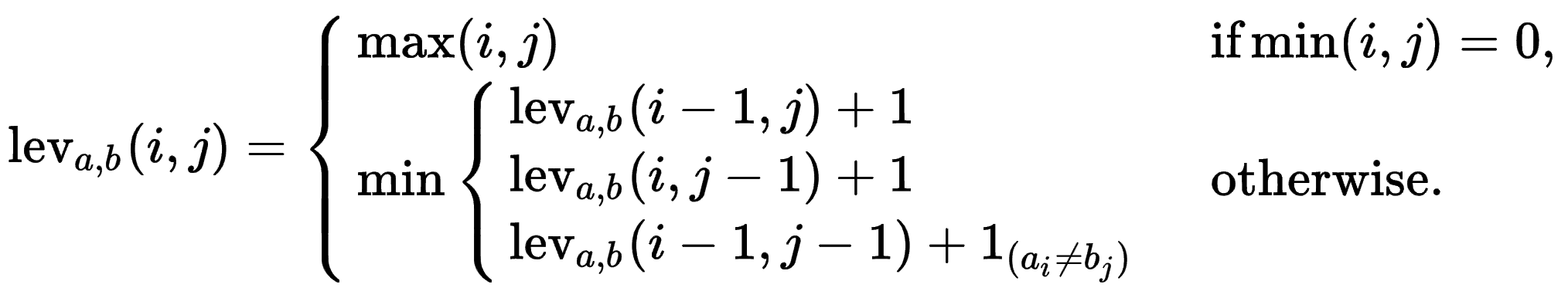### Levenshtein Distance

#### Recursive Implementation in C

``````
// len_s and len_t are the number of characters in string s and t respectively
int LevenshteinDistance(const char *s, int len_s, const char *t, int len_t)
{
int cost;

/* base case: empty strings */
if (len_s == 0) return len_t;
if (len_t == 0) return len_s;

/* test if last characters of the strings match */
if (s[len_s-1] == t[len_t-1])
cost = 0;
else
cost = 1;

/* return minimum of delete char from s, delete char from t, and delete char from both */
return minimum(LevenshteinDistance(s, len_s - 1, t, len_t    ) + 1,
LevenshteinDistance(s, len_s    , t, len_t - 1) + 1,
LevenshteinDistance(s, len_s - 1, t, len_t - 1) + cost);
}
``````

### Levenshtein Distance

#### Iterative Pseudocode Using Dynamic Programming

``````
function LevenshteinDistance(char s[1..m], char t[1..n]):
declare int v0[n + 1]
declare int v1[n + 1]

for i from 0 to n:
v0[i] = i

for i from 0 to m-1:
v1 = i + 1

for j from 0 to n-1:
if s[i] = t[j]:
substitutionCost := 0
else:
substitutionCost := 1
v1[j + 1] := minimum(v1[j] + 1, v0[j + 1] + 1, v0[j] + substitutionCost)

swap v0 with v1
return v0[n]
``````

### Search for PHP Implementation (1/2)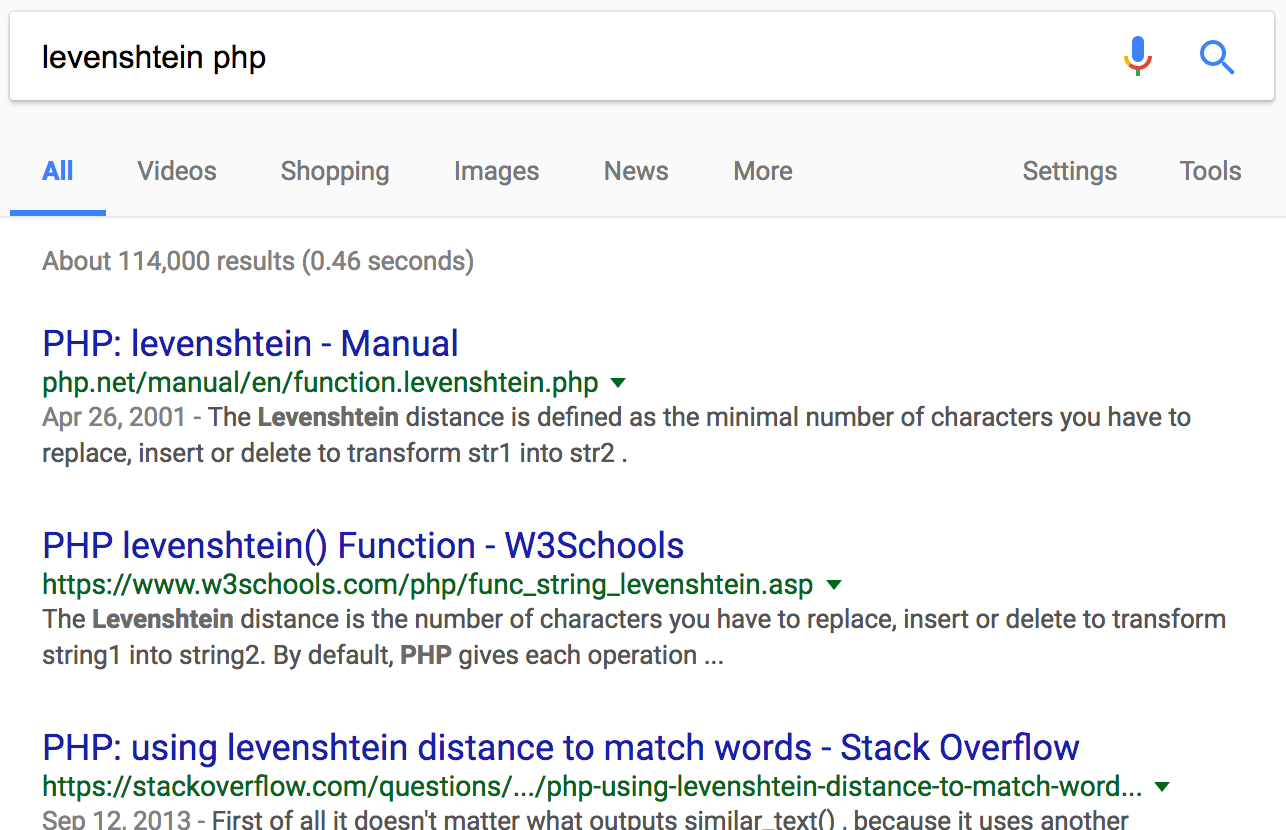### Search for PHP Implementation (2/2)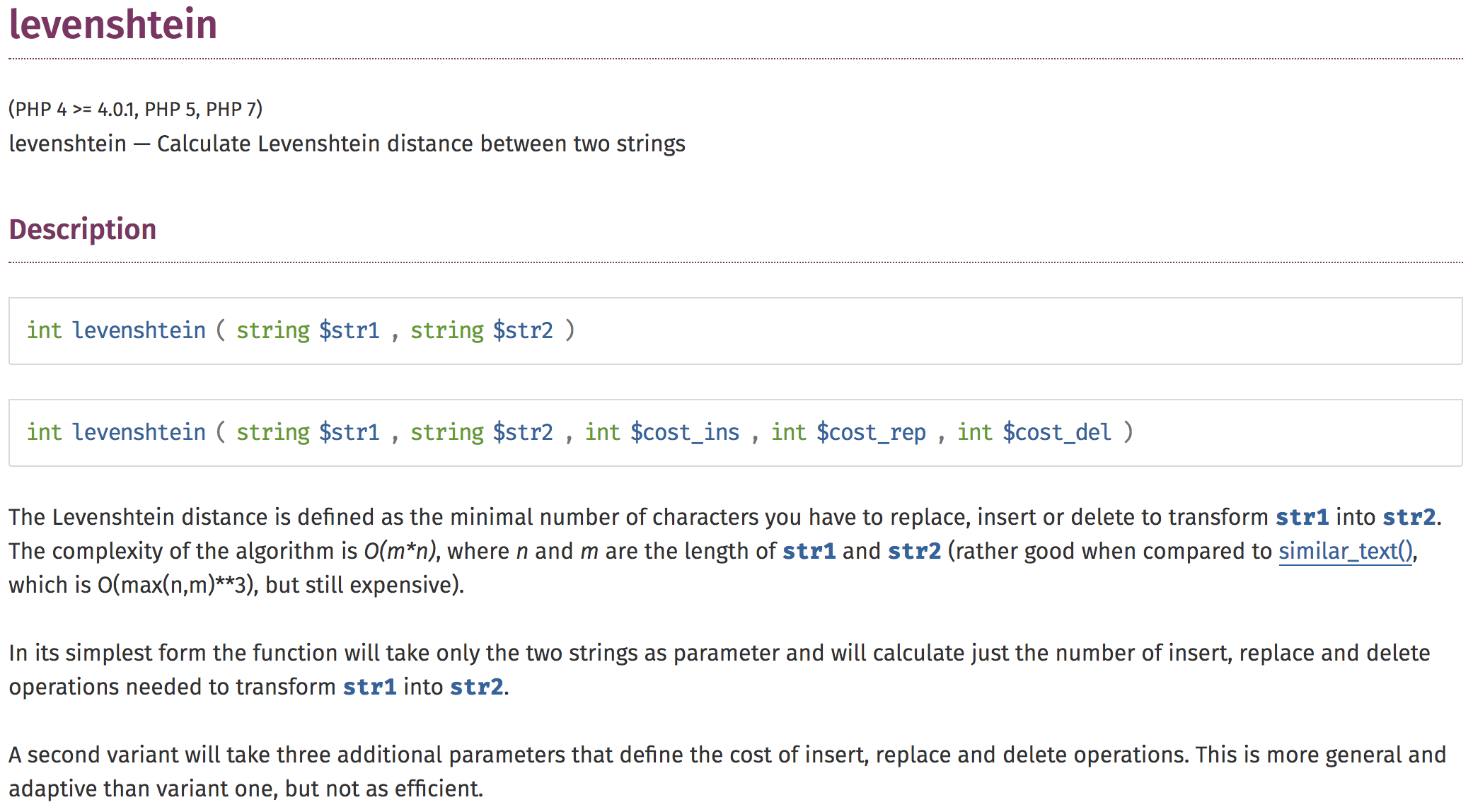### WP-CLI Implementation

``````
function get_suggestion( \$target, array \$options, \$threshold = 2 ) {
if ( empty( \$options ) ) {
return '';
}

// Calculate distances from each of known command strings to user entry.
foreach ( \$options as \$option ) {
\$distance = levenshtein( \$option, \$target );
\$levenshtein[ \$option ] = \$distance;
}

// Sort known command strings by distance to user entry.
asort( \$levenshtein );

// Fetch the closest command string.
reset( \$levenshtein );
\$suggestion = key( \$levenshtein );

// Only return a suggestion if below a given threshold.
return \$levenshtein[ \$suggestion ] <= \$threshold && \$suggestion !== \$target
? (string) \$suggestion
: '';
}
``````

# Questions ?I'm Alain Schlesser.